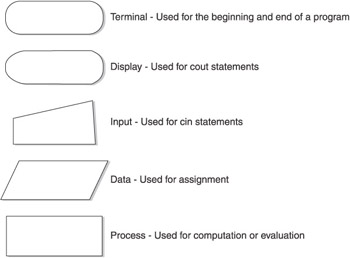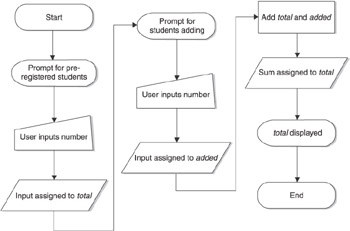# Flowcharting

## Flowcharting

A program, like a river , flows from beginning to end. Programmers may find it helpful, both in writing code and in understanding someone else s code, to visually depict the flow of the program. After all, as the adage goes, a picture is worth a thousand words. The ability to visualize the flow of a program becomes even more helpful as we transition from relatively simple programs that flow in a straight line to more complex varieties that branch in different directions based on the value of a relational expression.

Programmers use a flowchart to visually depict the flow of a program. Flowcharts use standardized symbols prescribed by the American National Standard Institute (ANSI), which prescribes other standards we will be using in this book. These flowcharting symbols represent different aspects of a program, such as the start or end of a program, user input, how it displays on a monitor, and so on. These symbols are joined by arrows and other connectors which show the connections between different parts of the program and the direction of the program flow. Figure 5-1 shows several commonly used flowchart symbols. Others will be introduced later in this book as they are used.Figure 5-1: Commonly used flowchart symbols

The following program from Chapter 4 can be depicted with a flowchart. As you may recall, this program first assigns to the integer variable total the value inputted by the user for the number of preregistered students. The program then assigns to the integer variable added the value inputted by the user for the number of students adding the course. The program then uses the addition operator to add two operands, total and added. The resulting sum is then assigned to total, which now reflects the total number of students in the course, both preregistered and added. That sum then is outputted.

` #include <iostream> using namespace std; int main(void) {  int total, added;  cout << "Enter number of pre-registered students: ";  cin >> total;  cout << "Enter number of students adding the course: ";  cin >> added;  total = total + added;  cout << "Total number of students: " << total;  return 0; } `

Figure 5-2 shows a flowchart of this program.This program was relatively linear. By contrast, the following programs will branch in different directions based on the value the user inputs. We will use flowcharts in later sections of this chapter to help explain how different code executes depending on the result of comparisons with the user s input.C++ Demystified(c) A Self-Teaching Guide
ISBN: 72253703
EAN: N/A
Year: 2006
Pages: 148